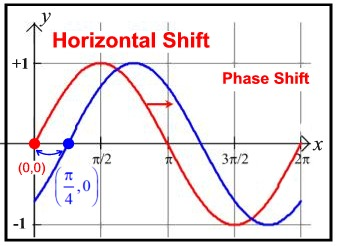Trigonometric Graphs Vocabulary MathBitsNotebook.com Terms of Use   Contact Person: Donna RobertsLet's see what vocabulary is needed to discuss the graphs of trigonometric functions.midline:

A midline of a sinusoidal function is the horizontal center line about which the function oscillates above and below. For y = sin x, the midline is y = 0 (the x-axis). The midline is parallel to the x-axis and is located half-way between the graphs maximum and minimum values.

The midline is affected by any vertical translations to the graph. For example, y = sin(x) + 2 has a midline at y = 2.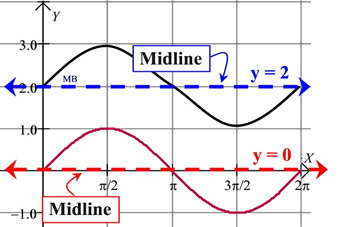amplitude: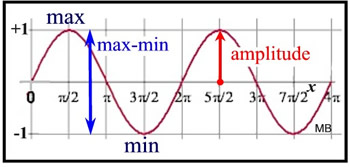The maximum of y = sin x is 1.
The minimum is -1.
The
amplitude is ½ (1 - (-1)) = 1.
The amplitude of a sinusoidal function is one-half of the positive difference between the maximum and minimum values of a function.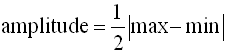It is simply the vertical distance from the x-axis (or midline) to the highest (or lowest) point on the curve. Amplitude is the magnitude (height) of the oscillation (wave) of a sinusoidal function.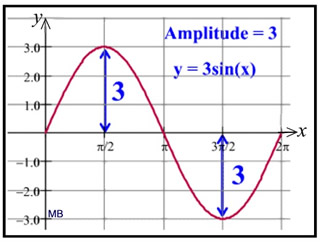maximum = +3, minimum = -3
amplitude = ½ (+3 - (-3)) = 3
As a distance measurement, amplitude is always positive.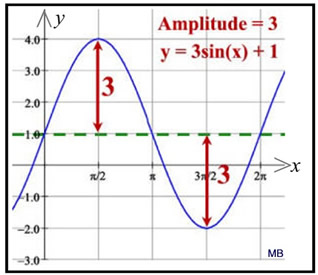maximum = +4, minimum = -2
amplitude= ½ (+4 - (-2)) = 3
Note: Look carefully at the two graphs shown above. At first glance they may look to be the same. But the graph on the right is a vertical shift of the graph on the left. While vertical shifts alter the maximum and minimum values of a function, they do not alter the amplitude. Also, horizontal shifts do not affect the amplitude.period:

A periodic function is a trigonometric function which repeats a pattern of y-values at regular intervals.  One complete repetition of the pattern is called a cycle.  The period of a function is the horizontal length of one complete cycle.

The period may also be described as the distance from one "peak" (max) to the next "peak" (max).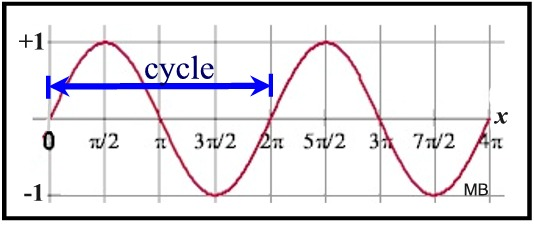This sine curve, y = sin x, has a period of 2π,
the horizontal length of one complete cycle.

In functional notation we could say:  The period is the smallest value of k in a function f for which there exists some constant k such that f (t) = f (t + k) for every number t in the domain of f.frequency:

The frequency of a trigonometric function is the number of cycles it completes in a given interval. This interval is generally 2π radians (or 360º) for the sine and cosine curves.
In terms of a formula: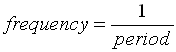It is also true that: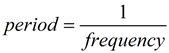This sine curve, y = sin x, completes 1 cycle in the interval from 0 to 2π radians. Its frequency is 1 in the interval of 2π.
FYI: In application problems, when frequency is measured "per second" it is called "Hertz"
(10 Hertz means 10 times per second).sinusoidal curve:

A sinusoidal curve is the graph of the sine function in trigonometry.

A sinusoid is the name given to any curve that can be written in the form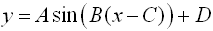The example at the right is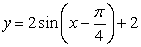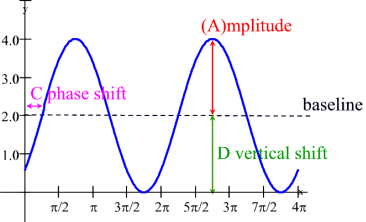The cosine wave is also said to be sinusoidal because of the relationship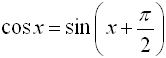which makes it the sine wave with a horizontal shift.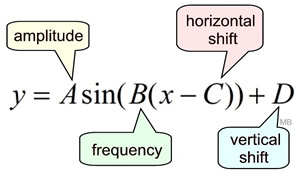The cosine function, which appears to have a "head start" on the sine function, is often said to lead the sine function (or the sine function lags the cosine function). Both sine and cosine fall under the category of sinusoids, since the cosine graph is simply a 90º shift to the left of the sine graph. The sine and cosine functions are the most popular of the trigonometric functions used for modeling.horizontal shift, phase shift: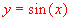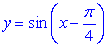From the sinusoidal equation,the horizontal shift is obtained by determining the change being made to the x-value.

The horizontal shift is C.

In mathematics, a horizontal shift of a trigonometric function may also be referred to as a phase shift.